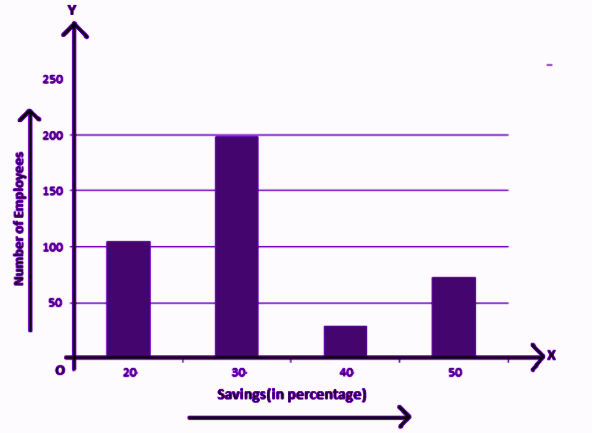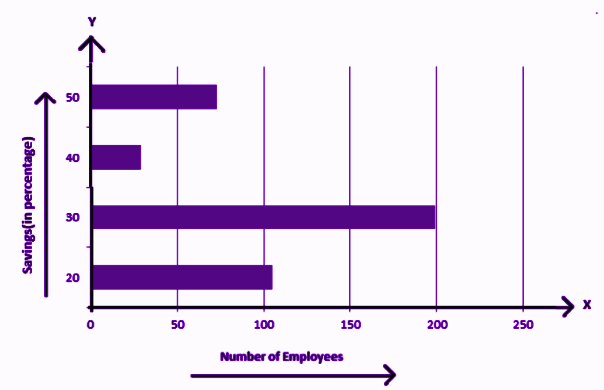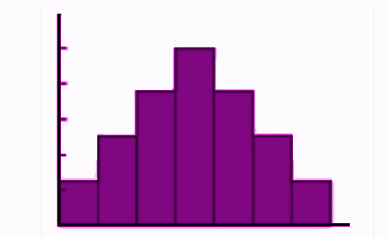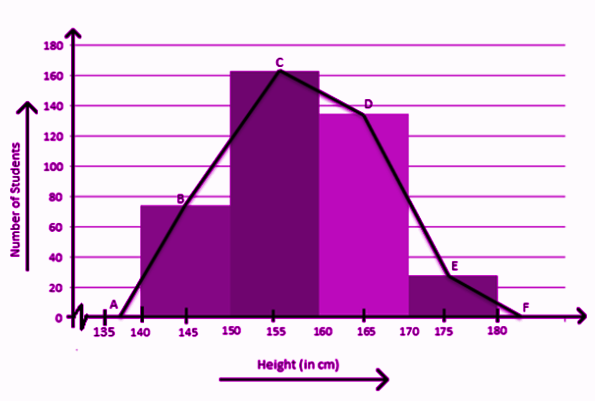Jet Set Go! All about Aeroplanes Jet Set Go! All about Aeroplanes

# Statistics For Class 9

Statistics for Class 9 Chapter 14 notes are made available here for students so that they can make use of it to prepare for exams. These notes consist of all the important topics covered in this chapter. Students who want to score good marks in Maths can refer to these notes.

Statistics are all about data. The data or numbers, which are collected with a definite purpose are called data. Data is the plural form of datum (the Latin word). The extraction of meaningful information from a given set of data is described under the branch of Mathematics called Statistics. It has its major applications in the real world where data is used to check the status of a business in the market. Both probability and statistics play a huge role in daily life such as in the field of Medicine, Engineering, Weather forecasting, etc.

## Statistics for Class 9 Notes

Let us learn here the important topics discussed in Maths Chapter 14 Statistics for Class 9 students. The list of topics is:

1. Collection of Data
2. Presentation of Data
3. Graphical representation of Data
4. Measures of Central Tendency

### Collection of Data Meaning

The meaning of a collection of data is to gather some sort of information to prepare a report or to do some analysis on them. Some examples are:

• Group of students under age 15 in a class.
• Height of 30 students in your class
• Number of family members in a house
• Age of the students in a class

### Presentation of Data

After collecting the data for a certain group, we have to now learn to present it. The presentation should be such that it should be meaningful, easily understood by everyone and the main features could be captured at a glance or by a single view. The important details should be highlighted properly. Here, to represent numerous data, we use a frequency distribution table, to condense the data into sub-groups. Let us see an example here.

Example: Suppose an exam was conducted for a class of 50 students. The marks obtained out of 100, by the students here are:

12, 23, 45, 55, 10, 33, 65, 78, 89, 22,

44, 55, 77, 88, 35, 65, 63, 61, 84, 89,

34, 27, 90, 65, 67, 45, 78, 98, 66, 77,

31, 41, 61, 68, 86, 34, 54, 59, 78, 89,

50, 29, 58, 63, 72, 87, 34, 65, 48, 91

Find how many students got more than 40 marks.

Solution: Let us arrange the data with respect to the marks obtained by the students.

 Marks Obtained Number of Students 0-20 2 21-40 10 41-60 11 61-80 17 81-100 10

Hence, from the above-grouped frequency distribution table, we can calculate the number of students who scored above 40 marks = 11+17+10 = 38

### Graphical Representation of Data

The grouped data of a collection of data can be represented using the graph as well. There are three ways by which we can represent the data in graphical form, which are;

1. Bar Graph
2. Histogram
3. Frequency Polygons

Bar Graph

A bar-graph gives a pictorial representation of data using vertical and horizontal rectangular bars, the length of the bars are proportional to the measure of data. Examples are:The above graph represents the data on the number of employees with respect to monthly salary savings. This is a vertical bar-graph, which could also be represented horizontally, such as;Histogram

A histogram can be defined as a set of rectangles with bases along with the intervals between class boundaries and with areas proportional to frequencies in the corresponding classes. Below is the general representation of a histogram.Frequency Polygon

A frequency polygon is used to compare sets of data or to show a cumulative frequency distribution. It utilises a line graph to express quantitative data.### Measures of central tendency

There are majorly three measures of central tendency:

• Mean
• Median
• Mode

Mean: Mean is the average of the given set of data.

x̄=∑ x/n

Where n is the number of observations

Median: The median is that value which divides the given number of observations into exactly two parts. First, the data set has to be arranged in an order, either ascending or descending. There are again two conditions here:

• If the number of observations is odd, then;

Median = [(n+1)/2]th observation or term

• If the number of observations is even, then the median will be mean of (n/2)th term and (n/2+1)th term.

Mode: The mode represents the frequently occurring value in the dataset.

Example: Find the mean, median and mode of the following data set.

2,3,6,7,4,5,3,8,3,9

Solution: Mean is the average of the given data;

x̄ = (2+3+6+7+4+5+3+8+3+9)/10 = 50/10 = 5

Now, to find the median, we need to arrange the data in ascending order.

2,3,3,3,4,5,6,7,8,9

Since, here the number of observations is even, therefore, the median will be the mean of the two middle terms.

Median = (4+5)/2 = 9/2 = 4.5

Mode = 3, since 3 is repeated here maximum number of times.

### Practice Questions

Q.1: Give one example of a condition in which:

(i) the mean is a proper measure of central tendency.

(ii) the mean is not a proper measure of central tendency but the median is a proper measure of central tendency.

Q.2: Find the mean, median mode of 14, 25, 14, 28, 18, 17, 18, 14, 23, 22, 14, 18.

Q.3: The relative humidity (in %) of a certain city for a month of 30 days was as follows:

98.1 98.6 99.2 90.3 86.5 95.3 92.9 96.3 94.2 95.1 89.2 92.3 97.1 93.5 92.7 95.1 97.2 93.3 95.2 97.3 96.2 92.1 84.9 90.2 95.7 98.3 97.3 96.1 92.1 89

(i) Write a grouped frequency distribution table with classes 90 – 95, 80 – 85, etc.

(ii) Which month or season do you think this data is about?

(iii) What is the range of this data?

(iv) Represent the data set using bar-graph and histogram